Target audience: You will want to know some basic probability.

Here's a moderately common problem: you make a bunch of observations of data, falling into some buckets or bins in a histogram, and maybe they have different weights too (because you know some observations will be more or less common than others in the real world, but they're not in your sampling, typically). You draw a histogram. Where do the error bars go?

Suppose you are taking N observations in total, and pick some particular bin, k. Let Sk be the sum of all weights of the observations/events lying in this bin,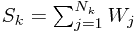Now here we have two sets of random variables - Nk is the number of observations lying in this bin, whilst Wj is the weight of each sample in the bin. We want to work out the variance of Sk.

Aside: As discussed in the paper mentioned below, it's actually debatable whether you should use this variance to obtain error bars. We'll focus here on the explicit problem of obtaining the variance of the above sum in terms of the exact distributions of the incoming data, and just assume we can approximate it in the usual ways given some data, and use this for error bars.

Here are two ways of looking at the problem:

## The Silly Way - Random Sums

The complicated way to do this involves using the so-called random sum formulae. It's surprisingly difficult to find things using this name online, so we'll go through the derivation of the relevant case. (You can look up Wald's Equation for more information.)

### Derivation of the random sum results

To make clear what we are doing, we assume that Xk for k = 1, 2, 3, ... are independent, identically distributed random variables with finite mean μ and finite variance σ2. Then we are given a random variable M with some finite mean and variance μM and σM2 and want to talk about the distribution of the sum of M of these variables.

Consider first the mean of the random sum X1 + ... + XM. This is pretty easy to work out using a so-called conditional expectation - the idea is that the expected value of an expression f(M,X1,...) depending on a random variable M can be worked out by first calculating the expected value F(m) of f given that M = m, and then working out the expectation of F(M), so long as M is independent of the other variables. (This is quite easy to prove, and known as the law of total expectation amongst other things.) Using this,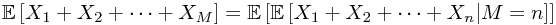But if we know how many terms there are in the sum, the expectation is easy to work out! We end up with just . But now we're done, because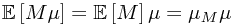... which is not a very flabbergasting result.

What about the variance? Let's start by attacking the expectation of the squared sum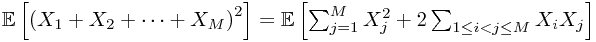and then using the same trick as before, we can write this as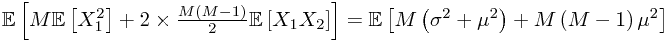where we've used the expression for the variance of X1 to work out the first term and the independence of these random variables to simplify the second term. Then, finally, we use similar formulae for M to recover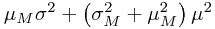From this, and the above expression for the expectation of the sum, we have our answer: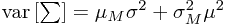which you probably wouldn't have guessed!

### Summary of random sum formulae

The two results we have proven are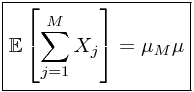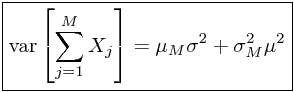Note that we can explicitly see here how the two sources of error (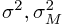) identified above contribute to the total error. We can deduce that error in the estimate of the weight dominates when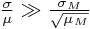and so on. (We will see below that the right-hand side is approximated by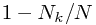for the histogram, where the last ratio is the probability of an event lying in the bin. Hence typically, when this ratio is fairly small, the larger source of error is determined by whether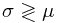.)

### Application to the histogram problem

In the histogram problem, we can easily come up with some estimators of μ, σ2, μN and σN2 and use these to estimate the variance of the above sum. The estimators of the means are simply the obvious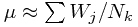and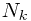. For the variance of the Wi, we can use the sample variance in the large Nk limit, which is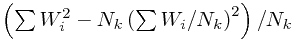. Finally, for the variance of Nk, we note that we want essentially the variance of the fraction Nk/N of data points in this bin, which suggests using the multinomial distribution, which would indicate the variance of Nk is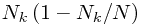.

Phew. Plugging this into the boxed formula above, we get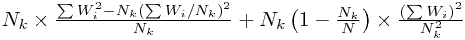... which simplifies to...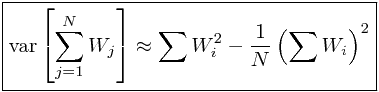... at which point a mathematician think something like "crap". Why? Because that formula is way too simple for that horrible mess to have been the best way to derive it! The particularly conspicuous thing about this equation is... can you see?

The thing that really strikes me, given how we got here, is that it doesn't mention Nk (except in the limits of the sum, but that somehow doesn't seem to count). So can we avoid this mess altogether?

## The Sensible Way

Well, we were led down the path of the above derivation by our idea that there are two sources of error in our bin total - how many samples ended up in it, and what the typical weight of a sample in that bin is. Can we avoid this division, given that it looks like we don't need to think like this?

Actually, yes we can, and it's so much simpler to think about it this way it makes the above look like a bad joke.

The message of the formula we found is that N, not Nk, is what describes 'how much data' we have collected in working out the bin total. So instead of thinking about our Nk weights Wj, let's think about N weights, Nk of which are the original Wj, and the rest of which are... zero!

This makes perfect sense, really - every sample from the original population contributes to the sum of weights in this bin, it's just that lots of them contribute zero. Sort of perverse, I guess, but it allows us to write the sum of weights in the bin as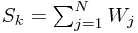where lots of the W'j happen to be zero. Why is this a good idea? Because we no longer have any mention of Nk, and more importantly no horrible random sums.

There's almost nothing to write, now - we have a sum of N random variables, and we want to estimate the variance. We already used the formula for that (in the case of estimating the mean of the Wj) - it's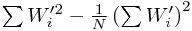... and when you remember that W'j = 0 except for the ones which fall in this bin, you realize we just got the answer. Already. Yep.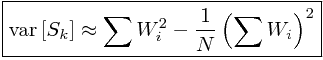And now you know.

## Equal weights case

The above all reduces, in the case Wj = 1, to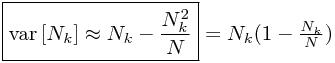as stated in the longer derivation - that is, it reduces to the multinomial distribution.

### Rare events - Poisson distribution

In accordance with the so-called law of small numbers or the law of rare events (or more formally, the Poisson limit theorem), if the probability of an event is very small, then the Poisson distribution approximates the binomial distribution corresponding to whether that event occurs. This manifests itself if we consider Nk/N small, as then the above variance is approximately Nk. This leads to the common practice in high energy physics etc. of plotting error bars which are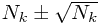.

See this blog (and the referenced paper) for discussions of the validity of using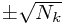error bars.

## Try it out

The following Mathematica code generates bigN (N above, and 10000 here by default) samples from a histogram bucket - the distribution given has 30% of data points in this bucket, with weights in a uniform [0,1] distribution.

It creates metaSamples such histograms, and then compares the actual standard deviation of the gathered distribution with the (average) estimate of the variance according to the above formula.

If you make metaSamples large enough - or just run the for loop repeatedly to gather more data - you should find the difference between the predicted error and observed error decaying to at least under 1%. (Of course, since N is finite, and so on, one does not expect exact agreement.)

meanDats = {};
varDats = {};

bigN = 10000;
metaSamples = 2500;
For[j = 1, j <= metaSamples, j++,
dat = RandomReal[{0, 1}, bigN];
dat = (Select[dat, (# >= 0.7) &] - 0.7)/0.3;
AppendTo[totDats, Total[dat]];
AppendTo[varDats, Total[dat^2] - Total[dat]^2/bigN];]

Print["Observed standand deviation of data: ",
StandardDeviation[meanDats]]
Print["Mean predicted error: ", Mean[varDats] // Sqrt]
Print["Percentage difference: ",
100*Abs[Sqrt[Mean[varDats]] - StandardDeviation[meanDats]]/
StandardDeviation[meanDats], "%"]
Print["Data points: ", Length[meanDats]]
Print["Standard deviation of predicted error: ",
StandardDeviation[
varDats // Sqrt], ", which is ", (StandardDeviation[
varDats // Sqrt]/(Mean[varDats] // Sqrt))*100, "% of the estimated error"]


After collecting 7500 (meta)samples, I obtained the following output:

Observed standand deviation of data: 27.9623
Mean predicted error: 27.8385
Percentage difference: 0.442649%
Data points: 7500
Standard deviation of predicted error: 0.260689, which is 0.936431% of the estimated error


So it certainly seems that this formula provides an excellent estimate of the error. Note that the predicted error from a single run deviates from the predicted error by less than 1%, so using this as an error estimate based on a single run also gives a very respectable error estimate.